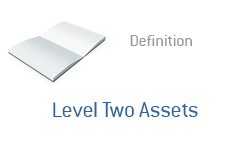## Definition of Level Two Assets

What are level two assets? What is the definition of a level 2 asset?

Alright, previously we have defined the terms "level 1 asset" and "level 3 asset", so now let's define a "level two asset".

A level one asset is an asset whose value can easily be determined through a quote on an active exchange. An example of a level one asset might be a publicly traded stock or a T-bill.

A level three asset is an asset whose value can NOT be easily determined. A level three asset is extremely illiquid and is not traded on any sort of an "active market". The value of a level three asset must be "guesstimated".

Example of level three assets include stakes in private companies and certain complex derivatives.A level two asset is not traded on an active exchange. The value of a level two asset can be determined through a quote on an inactive exchange, or through a model.

An example of a level two asset might be an interest rate swap or currency swap.

There is no "quoted" price for an interest rate swap, for example, but it's value can be determined through a simple model. That's a level two asset.

--

Davemanuel.com Articles That Mention Level Two Assets:

None